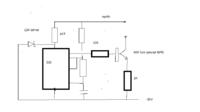# [SOLVED]Negative Constant Current Pulse Generation

Status
Not open for further replies.

#### amayilsamy

Dear friends,

How to generate rectangular negative constant current pulse for the load resistance 500 Ohm to 5 KOhm. I attached the image of wave form. How to generate this type wave form?

View attachment pulse.bmp

add a series resistor to your test resistor take the voltage drop accross it and feed it to a PWM so that the current accross the series resistor is constant.
Now you get a constant current pulsed output

A few parameters would be helpful, rise/fall/duration times of pulse, PRF, current, maximum voltage, output impedance?
Frank

A few parameters would be helpful, rise/fall/duration times of pulse, PRF, current, maximum voltage, output impedance?
Frank

Frequency= 1 Hz (.1 ms on , remain off)
Impedance = 0.5 K to 10 K
Current = 5 mA.

However the load resistance changes - within a 0.5 K to 10 K- the device is designed to monitor the output current drawn from it and will change its output voltage to keep the output current constant within a certain fixed range.

So with a max load of 5K and a current of 5 mA, this means the volt drop across the load will be 25V, so to allow a few volts across the supply, you need a supply of more then -30 V. The frequency seems trivial so we can ignore that. So starting off, going backwards. The output stage should be a N-P-N transistor, collector is your output terminal, earth is your other. The emitter goes to the -35V line via a 2K ohm resistor. The base is the input from a 555 timer. this needs to be run from a 12V line, so use the -35V as the earth line for the 555, run the 555 Vcc from the earth line via a 4K7 and a 12V zener from this junction to the -35V line. Do a search on Google (or here) for 555 astables, to find the resistor and capacitor values to give your required pulse waveform. take the output from the 555 to the base of the transistor via a 10K resistor. Sort of like this :-Frank

•amayilsamy

Points: 2